# Proportional relationship

The ordered pairs (6,24) and (1, s) represent a proportional relationship. Find the value of s.

Correct result:

s =  4

#### Solution: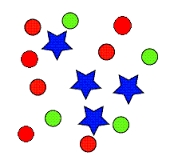We would be pleased if you find an error in the word problem, spelling mistakes, or inaccuracies and send it to us. Thank you!Tips to related online calculators
Need help calculate sum, simplify or multiply fractions? Try our fraction calculator.
Check out our ratio calculator.
Two vectors given by its magnitudes and by included angle can be added by our vector sum calculator.
Most natural application of trigonometry and trigonometric functions is a calculation of the triangles. Common and less common calculations of different types of triangles offers our triangle calculator. Word trigonometry comes from Greek and literally means triangle calculation.

## Next similar math problems:

• Segment in a triangle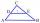In a triangle ABC with the side/AB/ = 24 cm is constructed middle segment/DE/ = 18 cm parallel to the side AB at a distance 1 cm from AB. Calculate the height of the triangle ABC to side AB.
• Sugar 9150 kg of sugar beet yields 24 kg of sugar. From how many tons of sugar beet can be obtained three tons of sugar?
• Set of coordinatesConsider the following ordered pairs that represent a relation. {(–4, –7), (0, 6), (5, –3), (5, 2)} What can be concluded of the domain and range for this relation?
• RatiosReduce the numbers: 50 in a 1:2 ratio 111 at a ratio of 2:3 70 at 10:50 560 at a ratio of 3:8
• CandiesThere are red, blue and green candies in bad. Red to green is in 6:11 ratio and blue to red in a 7: 5 ratio. In what proportion are blue to green candies?
• ApplesSchool kitchen bought 36kg apples for 12 kc/kg. How many kilograms of apples 1/4 cheaper can they buy for the same money?
• Everyone drinks the same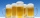24 bricklayers drink 72 beverage bottles a day at the construction site. How many bottles would 19 bricklayers need? Everyone drinks the same.
• Dried apples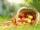How many kilograms of fresh apples id needed to 120 kg of dried apples when from 0.4 tons of fresh apples we get 75 kg of dried apples?
• Trapezoid - intersection of diagonalsIn the ABCD trapezoid is AB = 8 cm long, trapezium height 6 cm, and distance of diagonals intersection from AB is 4 cm. Calculate trapezoid area.
• Lookout towerCalculate the height of a lookout tower forming a shadow of 36 m if at the same time a column 2.5 m high has a shadow of 1.5 m.
• Hectares of forest12 workers plant 24 ha of forest in 6 days. In how many days will 15 people and 12 people plant the same area?
• Five combers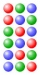Five combers harvest 12 rows of strawberries in 4 hours. How many rows of strawberries will two combers harvest in 10 hours?
• Metallurgical enterprise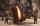Metallurgical company daily produces 318 tons of cast iron. How many tons of coke it burns to melt cast iron when 10 tons of cast consumes 6 tons of coke?
• Rails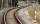18 m railway weighs 1260 kg. How much weighs 100 m of welded railways?
• Sixty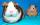Sixty percent of one-fifteen of the total is equal to thirty. What are two percent of the total?
• Proportion 3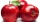For every 8 mango trees in the orchard, there are 4 star apple trees. If there are 1320 trees, how many trees of each kind are there?
• Gold inheritance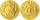The king divided the inheritance with his three sons in the ratio of 7: 6: 4. Two of them received 286,000 gold. How much each of the sons got.